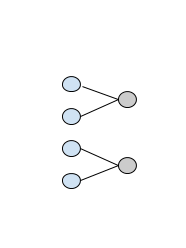# Network custom connections (paired connections) - performance issue

Hello,
I have a dataset repesented as Tensor of shape (x, y), where x are observations and y are features (y is around 5k). What I want to achieve is to have first layer where pairs of input features are connected to each neuron separately, as in this image:assuming the first layer (blue) is the input data and the second layer (gray) is the first hidden layer.
I would be able to follow the approach from Linear layer with custom connectivity (at least a similar one), but the problem is that I wanted to have ~2500 such neurons (or in another words, parallel/individual layers).
What I’ve come up with so far is the following (simplified case, but explains what I mean):

``````class CustomConnectivity(nn.Module):
def __init__(self):
self.indexer = [Variable(torch.LongTensor([i, i+1])) for i in range(0, 5000, 2)]
self.linear_list = nn.ModuleList([nn.Linear(2, 1) for _ in range(2500)])
self.fc_last = nn.Linear(2500, 1)

def forward(self, x):
lin_pre_outputs = [F.relu(self.linear_list[i](x.index_select(dim=1, index=self.indexer[i]))) for i in range(2500)]
x = torch.cat(lin_pre_outputs, dim=1)
x = F.sigmoid(self.fc_last(x))
return x

``````

This is the fastest approach I could come up with, but unfortunately it does not run fast at all. The approaches with similar number of parameters seem to run 20-30x faster than this. This network is actually faster to train on CPU than on GPU, which I guess is the problem of input indexing and/or the for loop.
I tried the approach with creating fully connected later with zero’d weights and gradients, but despite of being much much uglier, it doesn’t really run faster and is much more memory consuming. I failed to implement some kind of sparse tensor approach (the API is undocumented).

What do you think is the most efficient (mostly computationally efficient, memory efficiency is less important) way to implement such network?

because of modern CPUs / GPUs, it’s easier to zero-out particular weights of a large Linear layer and do a dense matrix multiply, than do what you are doing (keep a linear_list of 2500 very tiny and serially executed matrix multiplies)

Yeah I am aware of that, the problem is that fully connected layer + mask are both big matrices and I ran into memory issues on my GPU. I was rather thinking whether there is faster indexing procedure than the one I’m using.
Is a masking/zeroing approach implementation available somewhere so I could refer to it and look for potential mistakes in my code?

Hi,
have you been able to solve the problem with low performance when using a custom connected layers? I am trying to do something similar but I am afraid that if I have used dense layers with masks, I would not be able to fit the model into the GPU’s memory.

Nope. I tried both approaches, the zeroing-out mask and a list/loop of small fully connected layers. The former did not fit into my GPU (I was not able to handle this with sparse matrices as there were not enough documentation of these) while the latter worked terribly slowly.
I created another topic asking for help with implementation Gradient masking in register_backward_hook for custom connectivity - efficient implementation, but I did not get any.